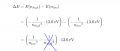# Calculate the energy of the Photon

#### AlbertHall

Joined Jun 4, 2014
11,226
For the n=1 to n=3 transition the photon must supply the difference in energy between the two levels.
For the second case the final energy is just the kinetic energy as the electron is not part of the atom.

•Mojo Pin__

#### Mojo Pin__

Joined Apr 13, 2019
83
For the n=1 to n=3 transition the photon must supply the difference in energy between the two levels.
For the second case the final energy is just the kinetic energy as the electron is not part of the atom.

Hi! Thanks for the responsewhen trying to find the answer I thought it might be something to do with the image i posted below.

For transition 2 I thought that because the electron starts at energy level 3 and then is ejected there is only:

1/nlow^2

because there is no higher energy level I ignore the:

-1/nhigh^2

but include kinetic energy to calculate the energy of photon 2.

Do you think this would be an acceptable way of thinking about it?

Cheers!